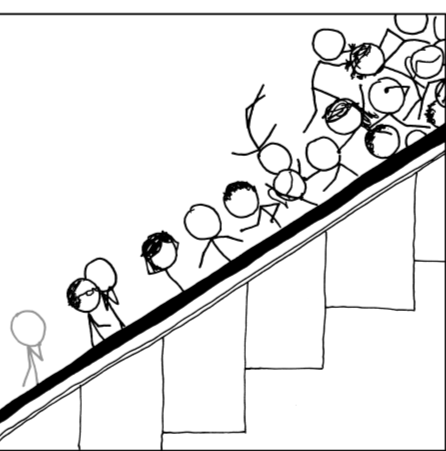You are currently browsing the tag archive for the ‘Gross-Pitaevskii hierarchy’ tag.$\displaystyle i \hbar \partial_t |\psi \rangle = H |\psi\rangle$

is the fundamental equation of motion for (non-relativistic) quantum mechanics, modeling both one-particle systems and${N}$-particle systems for${N>1}$. Remarkably, despite being a linear equation, solutions${|\psi\rangle}$ to this equation can be governed by a non-linear equation in the large particle limit${N \rightarrow \infty}$. In particular, when modeling a Bose-Einstein condensate with a suitably scaled interaction potential${V}$ in the large particle limit, the solution can be governed by the cubic nonlinear Schrödinger equation$\displaystyle i \partial_t \phi = \Delta \phi + \lambda |\phi|^2 \phi. \ \ \ \ \ (1)$

I recently attended a talk by Natasa Pavlovic on the rigorous derivation of this type of limiting behaviour, which was initiated by the pioneering work of Hepp and Spohn, and has now attracted a vast recent literature. The rigorous details here are rather sophisticated; but the heuristic explanation of the phenomenon is fairly simple, and actually rather pretty in my opinion, involving the foundational quantum mechanics of${N}$-particle systems. I am recording this heuristic derivation here, partly for my own benefit, but perhaps it will be of interest to some readers.

This discussion will be purely formal, in the sense that (important) analytic issues such as differentiability, existence and uniqueness, etc. will be largely ignored.

### Recent CommentsVincent on A function field analogue of R…Vincent on A function field analogue of R…christangdt on Analysis ITerence Tao on The alternative hypothesis for…imran saleh on Learn the limitations of your…Anonymous on The alternative hypothesis for…Anonymous on A function field analogue of R…Terence Tao on A function field analogue of R…Terence Tao on Analysis Ichristangdt on Analysis Ishabiatiya on A function field analogue of R…Will Sawin on A function field analogue of R…Terence Tao on A function field analogue of R…Will Sawin on A function field analogue of R…Will Sawin on A function field analogue of R…